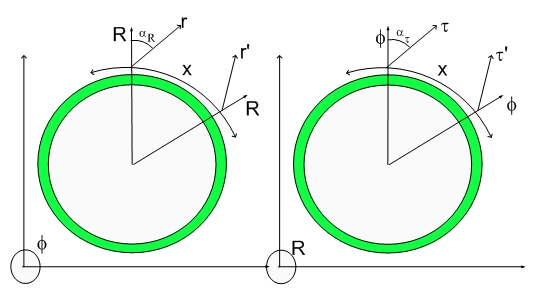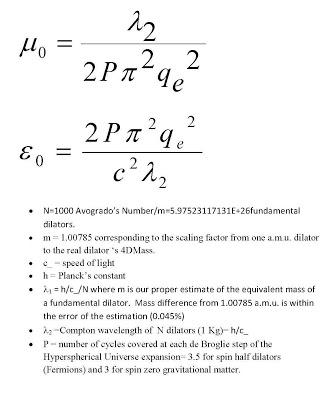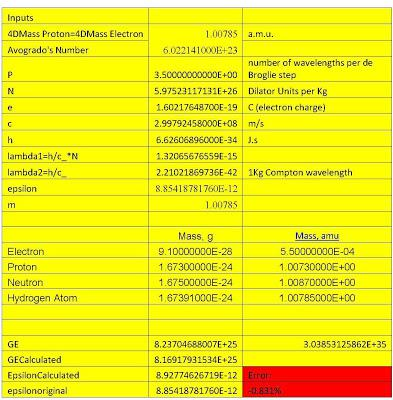# Hypergeometrical Universe EpsilonHow to calculate Cosmological Constants

The vacuum permitivity is a Cosmological Constant, that is, there is NO formula for it. That might be the case in other theories. In mine, there is a simple formula for both the vacuum permitivity and the magnetic susceptibility and they obey the standard formula to yield the speed of light....:)

This might be the simplest way I can use to convey that this theory is simple but as you might know, it is easy to shuffle equations to yield a new equation. It is very difficult to simplify things...:)The calculated vacuum permitivity and magnetic susceptiblity error is zero since we used it to obtain the best estimate of the electron (proton) 4DMass. The very small discrepancy (0.8%) is attributed at this time to the inherent anisotropy of the hyperspherical expansion, the inherent error associated with the assumption that one is at rest with respect to the Fabric of Space...:)

I was certainly made a believer when I obtained this number... There is no theory that can calculate these two cosmological constant (electrostatic permitivity and the magnectic susceptibility) , even less with such precision and ease....:)

Of course, this formulas are in the new version of the
paper.

Cheers,

MP
Currently unrated

### New Comment

required

required (not published)

optional

required